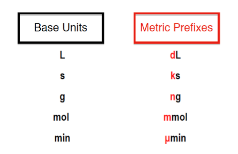Clutch Prep is now a part of Pearson
Ch.1 - Intro to General ChemistryWorksheetSee all chapters

# Metric Prefixes

See all sections
Sections
Classification of Matter
Physical & Chemical Changes
Chemical Properties
Physical Properties
Intensive vs. Extensive Properties
Temperature
Scientific Notation
SI Units
Metric Prefixes
Significant Figures
Significant Figures: Precision in Measurements
Significant Figures: In Calculations
Conversion Factors
Dimensional Analysis
Density
Density of Geometric Objects
Density of Non-Geometric Objects

Metric Prefixes are “labels” that can be placed in front of base units.

###### Metric Prefix Multipliers

Concept #1: Understanding Metric Prefixes

Concept #2: Using the Metric Prefix ChartExample #1: Convert the following value to the desired units: 694 kg to µg

Practice: Which quantity in the following pair is smaller?

Practice: Use the prefix multipliers to express each measurement without any exponents.

a) 32 x 10-13 L

b) 7.3 x 106

c) 18.5 x 1011 s

Practice: Use scientific notation to express each quantity with only the base unit.

a) 83 µm

b) 193 kg

c) 2.7 mmol

Practice: If a room has a volume of 1.15 x 108 cm3, what is the volume in km3?